Courses

# Thermodynamics, General Terms and State Variables Class 11 Notes | EduRev

## JEE : Thermodynamics, General Terms and State Variables Class 11 Notes | EduRev

The document Thermodynamics, General Terms and State Variables Class 11 Notes | EduRev is a part of the JEE Course Chemistry Class 11.
All you need of JEE at this link: JEE

INTRODUCTION
Thermodynamics is a Greek word. Its literal meaning is motion or flow (dynamics) of heat (thermos).

DEFINITION
Thermodynamics, science of the relationship between heat, work, temperature, and energy. In broad terms, thermodynamics deals with the transfer of energy from one place to another and from one form to another.
The key concept is that heat is a form of energy corresponding to a definite amount of mechanical work.
Thermodynamics deals with energy interaction b/w two bodies & its effect on the properties of matter.

SCOPE OF THERMODYNAMICS

• Feasibility of a process: It helps to lay down the criteria for predicting feasibility or spontaneity of a process, including a chemical reaction, under a given set of conditions.
• Extent of a process: It helps to determine the extent to which a process, including a chemical reaction, can proceed before attainment of equilibrium.

Efficiency of a process

• The study of thermodynamics is based on three generalizations derived from experimental results. These generalizations are known as first, second and third law of thermodynamics based on human experience and there is no formal proof for them.
• Laws of thermodynamics apply only when a system is in equilibrium or moves from one equilibrium state to another equilibrium state.

LIMITATIONS OF THERMODYNAMICS

• The laws of thermodynamics deal with energy changes of macroscopic systems involving a large number of molecules rather than microscopic systems containing a few molecules.
• Thermodynamics is not concerned about how and at what rate these energy transformations are carried out, but is based on initial and final states of a system undergoing the change.

TERMS USED IN THERMODYNAMICS

System + Boundary + Surrounding = Universe

(i) System: The part of the universe under thermodynamic observation is called system.
(ii) Surroundings: All the part of the universe excepting system is called surroundings.
(iii) Boundary: The part which separates system and surroundings is called boundary it may be rigid or flexible.

• It may be diathermic (Heat can be exchanged) or adiabatic.
• The boundary may be real or imaginary; it may be rigid or non -rigid; it may be a conductor or a non-conductor of heat.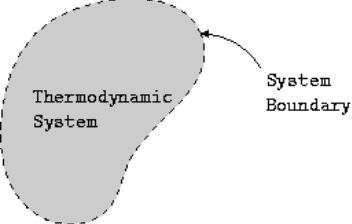Fig:  System Thermodynamic

TYPES OF SYSTEMS
(i) Open system: The system which can exchange matter as well as energy with the surroundings.
For example, a liquid kept in an open flask.
(ii) Closed System: A system which can exchange only energy with the surroundings.
For example, a liquid in a closed vessel.
(iii) Isolated System: A system which can neither exchange matter nor energy with the surroundings.
For example, a thermally insulated flask.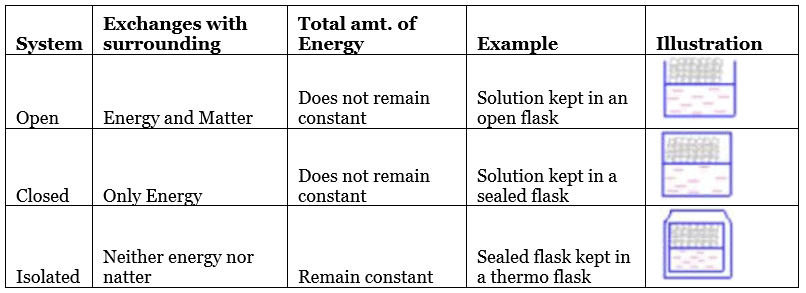STATE OF A SYSTEM
When macroscopic properties of a system have definite values, the system is said to be in definite state. Whenever there is a change in any one of the macroscopic properties, the system is said to change into a different state. Thus, the state of a system is fixed by its macroscopic properties.

STATE VARIABLES
State or condition of a system is described by certain measurable properties & these measurable properties are called state variables. State variables or the thermodynamics parameters depends only upon the initial and final states of the system and independent of the manner as to how the change is brought are called state functions. Some common state functions are internal energy, enthalpy, entropy, free energy, pressure, temperature, volume etc.

STATE FUNCTIONS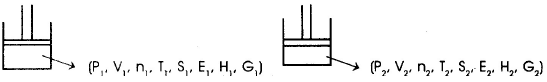State function depends only on initial & final state of the system. If does not depend on the path or how process was carried out.

Example: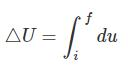Where ΔU = uf - u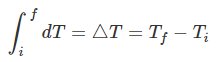Solution. The parameters which are required to completely define the state of the system are called state functions.
State functions are path independent function.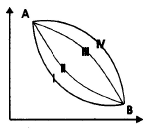• Process-1  ΔP = ΔP ⇐ Process - II
• Process-1 ⇒ ΔV = ΔV ⇐ Process - II
• Process-1 ⇒ Δn = Δn ⇐ Process - II
• Process-1 ⇒ ΔT = ΔT ⇐ Process - II
• Process-1 ⇒ ΔE = ΔE ⇐ Process - II
• Process-1 ⇒ ΔH = ΔH ⇐ Process - II
• Process-1 ⇒ ΔG = ΔG ⇐ Process - II
• Process-1 ⇒ ΔS = ΔS ⇐ Process - II

Path independent means the difference in state functions will be same for any path followed between two states.

Path Functions: Path function depends on the initial as well as final state of a system & also depends on the path of the process.
Example: heat and work.

EXTENSIVE AND INTENSIVE PROPERTIES
An extensive property of a system is that which depends upon the amount of the substance present in the system.
Example: mass, volume and energy.
An intensive property of a system is that which is independent of the amount of the substance present in the system.
Example: Temperature, pressure, density, concentration, viscosity, surface tension, refractive index etc.

INTENSIVE PROPERTIES VERSUS EXTENSIVE PROPERTIES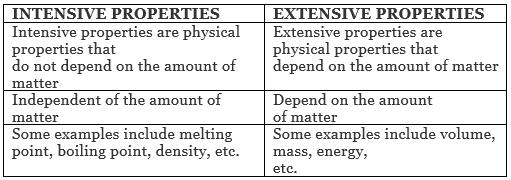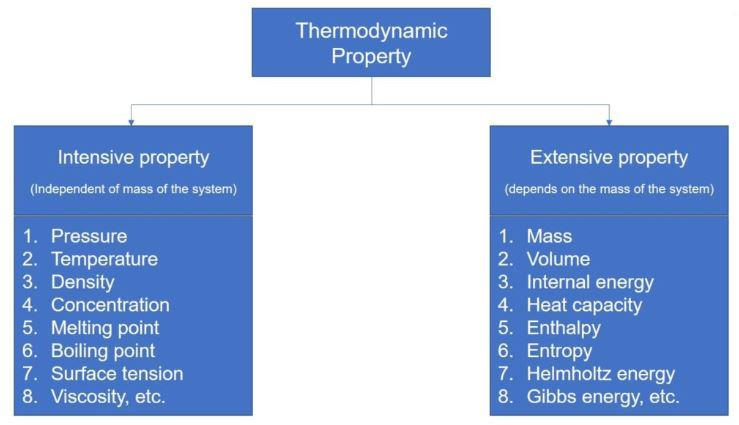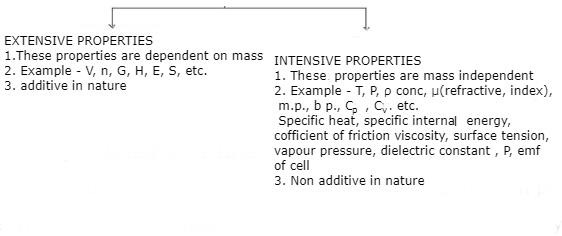How to identify extensive or intensive properties?
If a system in a particular state is divided into two equal or unequal parts, the properties which have value equal to the original value of that property for the whole of the system is called an Intensive property. While the properties which have values different from the values for whole of the system are called extensive properties.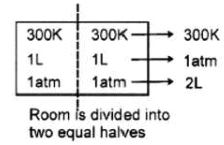For example: Consider air in a room at temp of 300K, 1 atm pressure. Now, if the room is divided by some boundary( imaginary or real) into two parts( equal or unequal) then in these two parts : The temperature, pressure, density of the gas, concentration of gaseous molecules etc. will have the same value as that of for whole of the system. (intensive) While the volume of two parts, mass of gas in two parts, total energy of the gaseous molecules in the two parts, entropy the two parts etc. will be different from the values of these properties as for the whole of the system initially. (extensive)

• Ratio of two extensive properties is an intensive property.
Example: Density = Mass/Volume
Offer running on EduRev: Apply code STAYHOME200 to get INR 200 off on our premium plan EduRev Infinity!

,

,

,

,

,

,

,

,

,

,

,

,

,

,

,

,

,

,

,

,

,

,

,

,

;